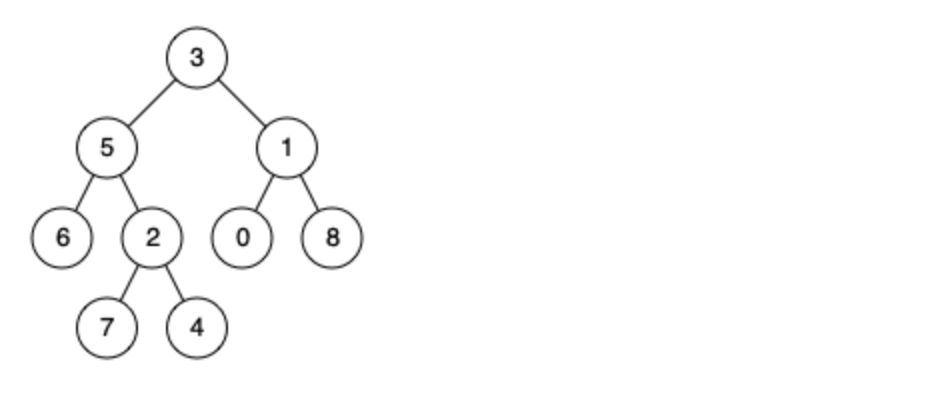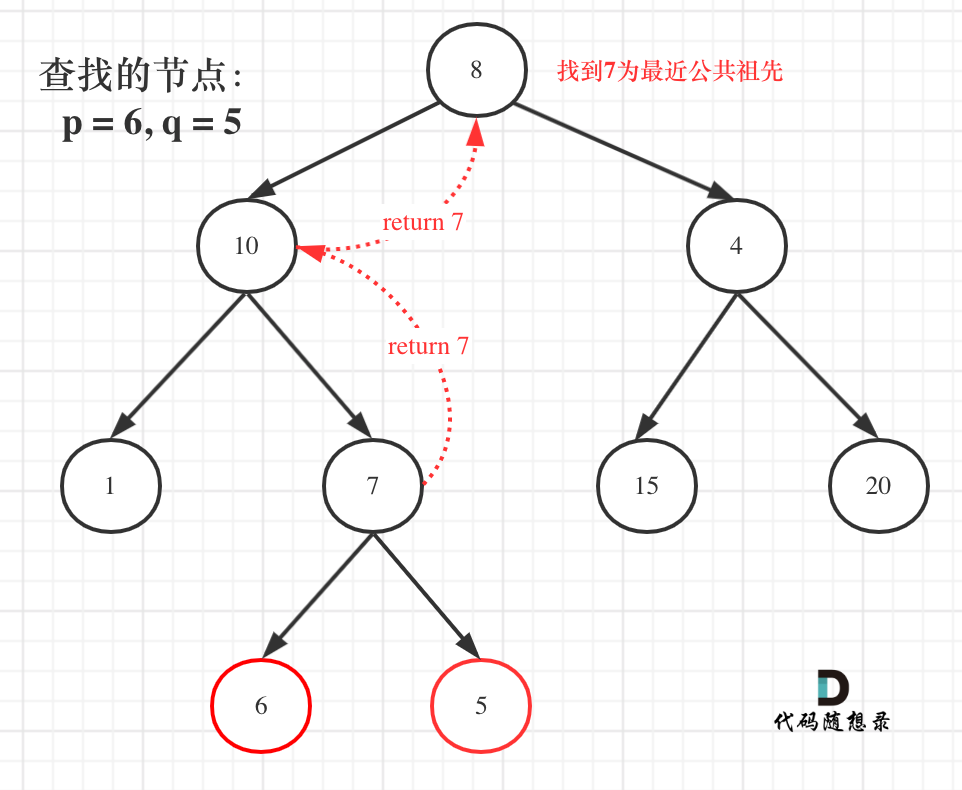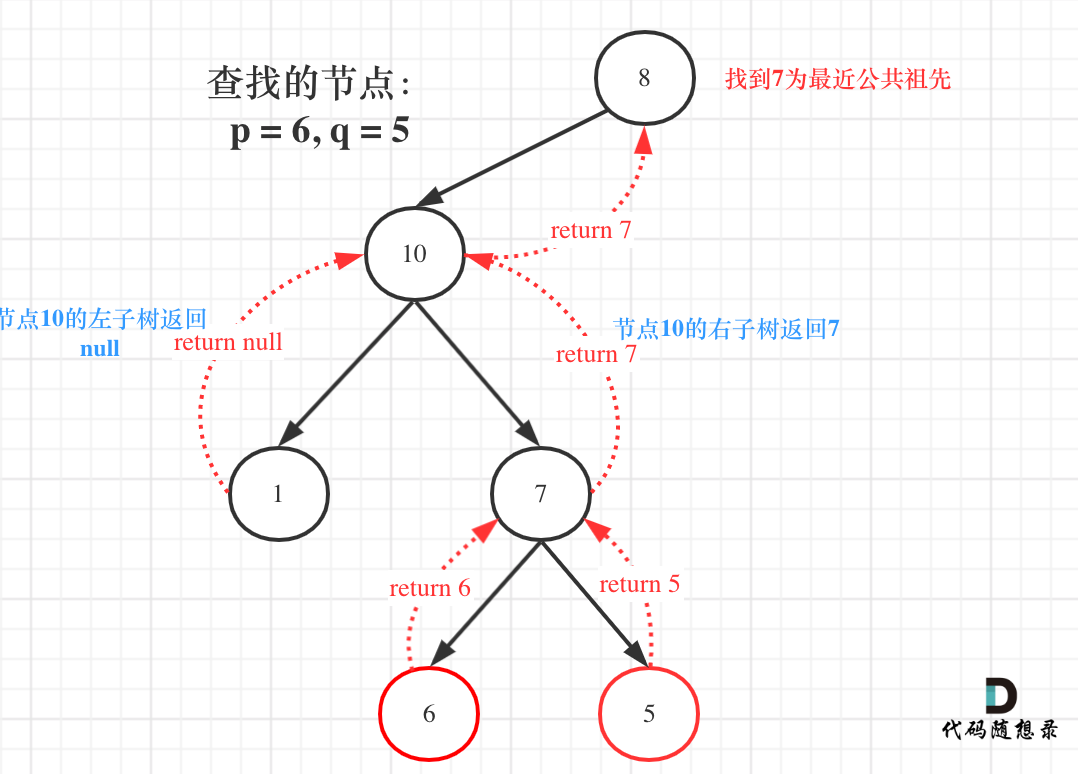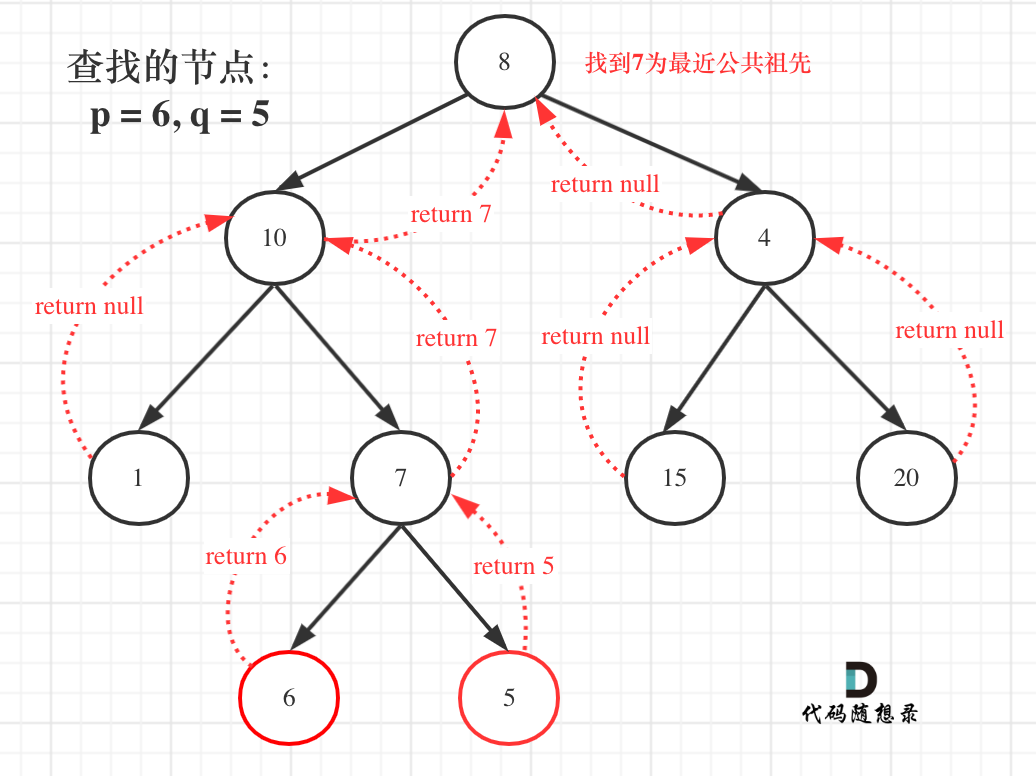# # 236. 二叉树的最近公共祖先• 所有节点的值都是唯一的。
• p、q 为不同节点且均存在于给定的二叉树中。

# # 思路

• 确定递归函数返回值以及参数

``````TreeNode* lowestCommonAncestor(TreeNode* root, TreeNode* p, TreeNode* q)
``````
1
• 确定终止条件

``````if (root == q || root == p || root == NULL) return root;
``````
1
• 确定单层递归逻辑

``````if (递归函数(root->left)) return ;

if (递归函数(root->right)) return ;
``````
1
2
3

``````left = 递归函数(root->left);
right = 递归函数(root->right);
left与right的逻辑处理;
``````
1
2
3``````left = 递归函数(root->left);
right = 递归函数(root->right);
left与right的逻辑处理;
``````
1
2
3

``````TreeNode* left = lowestCommonAncestor(root->left, p, q);
TreeNode* right = lowestCommonAncestor(root->right, p, q);

``````
1
2
3``````if (left == NULL && right != NULL) return right;
else if (left != NULL && right == NULL) return left;
else  { //  (left == NULL && right == NULL)
return NULL;
}

``````
1
2
3
4
5
6``````class Solution {
public:
TreeNode* lowestCommonAncestor(TreeNode* root, TreeNode* p, TreeNode* q) {
if (root == q || root == p || root == NULL) return root;
TreeNode* left = lowestCommonAncestor(root->left, p, q);
TreeNode* right = lowestCommonAncestor(root->right, p, q);
if (left != NULL && right != NULL) return root;

if (left == NULL && right != NULL) return right;
else if (left != NULL && right == NULL) return left;
else  { //  (left == NULL && right == NULL)
return NULL;
}

}
};
``````
1
2
3
4
5
6
7
8
9
10
11
12
13
14
15
16

``````class Solution {
public:
TreeNode* lowestCommonAncestor(TreeNode* root, TreeNode* p, TreeNode* q) {
if (root == q || root == p || root == NULL) return root;
TreeNode* left = lowestCommonAncestor(root->left, p, q);
TreeNode* right = lowestCommonAncestor(root->right, p, q);
if (left != NULL && right != NULL) return root;
if (left == NULL) return right;
return left;
}
};
``````
1
2
3
4
5
6
7
8
9
10
11

# # 总结

1. 求最小公共祖先，需要从底向上遍历，那么二叉树，只能通过后序遍历（即：回溯）实现从低向上的遍历方式。

2. 在回溯的过程中，必然要遍历整棵二叉树，即使已经找到结果了，依然要把其他节点遍历完，因为要使用递归函数的返回值（也就是代码中的left和right）做逻辑判断。

3. 要理解如果返回值left为空，right不为空为什么要返回right，为什么可以用返回right传给上一层结果。

# # 其他语言版本

## # Java

``````class Solution {
public TreeNode lowestCommonAncestor(TreeNode root, TreeNode p, TreeNode q) {
if (root == null || root == p || root == q) { // 递归结束条件
return root;
}

// 后序遍历
TreeNode left = lowestCommonAncestor(root.left, p, q);
TreeNode right = lowestCommonAncestor(root.right, p, q);

if(left == null && right == null) { // 若未找到节点 p 或 q
return null;
}else if(left == null && right != null) { // 若找到一个节点
return right;
}else if(left != null && right == null) { // 若找到一个节点
return left;
}else { // 若找到两个节点
return root;
}
}
}

``````
1
2
3
4
5
6
7
8
9
10
11
12
13
14
15
16
17
18
19
20
21
22

## # Python

``````class Solution:
"""二叉树的最近公共祖先 递归法"""

def lowestCommonAncestor(self, root: 'TreeNode', p: 'TreeNode', q: 'TreeNode') -> 'TreeNode':
if not root or root == p or root == q:
return root

left = self.lowestCommonAncestor(root.left, p, q)
right = self.lowestCommonAncestor(root.right, p, q)

if left and right:
return root
if left:
return left
return right
``````
1
2
3
4
5
6
7
8
9
10
11
12
13
14
15

## # Go

``````func lowestCommonAncestor(root, p, q *TreeNode) *TreeNode {
// check
if root == nil {
return root
}
// 相等 直接返回root节点即可
if root == p || root == q {
return root
}
// Divide
left := lowestCommonAncestor(root.Left, p, q)
right := lowestCommonAncestor(root.Right, p, q)

// Conquer
// 左右两边都不为空，则根节点为祖先
if left != nil && right != nil {
return root
}
if left != nil {
return left
}
if right != nil {
return right
}
return nil
}
``````
1
2
3
4
5
6
7
8
9
10
11
12
13
14
15
16
17
18
19
20
21
22
23
24
25
26

## # JavaScript

``````var lowestCommonAncestor = function(root, p, q) {
// 使用递归的方法
// 需要从下到上，所以使用后序遍历
// 1. 确定递归的函数
const travelTree = function(root,p,q) {
// 2. 确定递归终止条件
if(root === null || root === p||root === q) {
return root;
}
// 3. 确定递归单层逻辑
let left = travelTree(root.left,p,q);
let right = travelTree(root.right,p,q);
if(left !== null&&right !== null) {
return root;
}
if(left ===null) {
return right;
}
return left;
}
return  travelTree(root,p,q);
};
``````
1
2
3
4
5
6
7
8
9
10
11
12
13
14
15
16
17
18
19
20
21
22

## # TypeScript

``````function lowestCommonAncestor(root: TreeNode | null, p: TreeNode | null, q: TreeNode | null): TreeNode | null {
if (root === null || root === p || root === q) return root;
const left = lowestCommonAncestor(root.left, p, q);
const right = lowestCommonAncestor(root.right, p, q);
if (left !== null && right !== null) return root;
if (left !== null) return left;
if (right !== null) return right;
return null;
};
``````
1
2
3
4
5
6
7
8
9

@2021-2022 代码随想录 版权所有 粤ICP备19156078号